# DAV Class 5 Maths Chapter 12 Worksheet 1 Solutions

The DAV Books Solutions Class 5 Maths and DAV Class 5 Maths Chapter 12 Worksheet 1 Solutions of Percentage offer comprehensive answers to textbook questions.

## DAV Class 5 Maths Ch 12 Worksheet 1 Solutions

Question 1.
Fill in the boxes.
(a)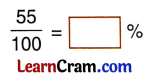Solution:
$$\frac{55}{100}$$ = 55

(b)Solution:
$$\frac{4}{100}$$ = 4(c)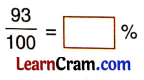Solution:
$$\frac{93}{100}$$ = 93

(d)Solution:
$$\frac{125}{100}$$ = 125

(e)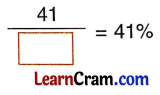Solution:
$$\frac{41}{100}$$ = 41%

(f)Solution:
$$\frac{11}{100}$$ = 11%

(g)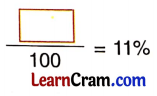Solution:
$$\frac{11}{100}$$ = 11%(h)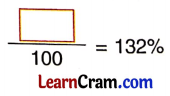Solution:
$$\frac{132}{100}$$ = 132%

Question 2.
Solve the following questions.
(a) In a box, there are 100 chalks. Out of these 65 chalks are blue and the rest are pink in colour. Complete the following statements.Solution:
Fraction of blue chalks = $$\frac{65}{100}$$ = 65%
Number of pink chalks = 35
Fraction of pink chalks = $$\frac{35}{100}$$ = 35%

(b) Anuj had 100 stamps. Out of these, 32 are foreign stamps and the rest are Indian stamps. Find the percentage of Indian stamps that Anuj had.
Solution:
% of Indian stamps = ?
Foreign stamps = 32
No, of Indian stamps = 100 – 32 = 68
% of Indian stamps = 68%(c) Here is a big square divided into 100 small squares. Five squares are shaded black to show 5%.Now shade the squares to represent the following percent.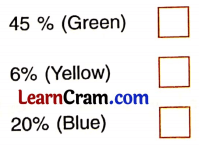Solution: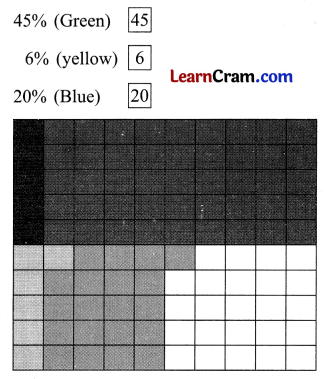DAV Class 5 Maths Chapter 12 Worksheet 1 Notes

Fractions whose denominator is equal to hundred is called percentage.
e.g., $$\frac{52}{100}$$, $$\frac{33}{100}$$

Cent means 100. ‘Percent’ means ‘for every 100’.

By two methods a fraction can be converted into a percentage:

• By converting a fraction into an equivalent fraction with a denominator of 100.
e.g., $$\frac{7}{25}=\frac{7 \times 4}{25 \times 4}=\frac{28}{100}$$ = 28%
• By multiplying the fraction by 100.
e.g., $$\frac{7}{25}$$ = $$\frac{7}{25}$$ × 100 = $$\frac{700}{25}$$ = 28%

By two methods a decimal can be converted into a percentage:

• By converting decimals into equivalent fractions with denominator 100.
e.g., 0.4 = $$\frac{4 \times 10}{10 \times 10}=\frac{40}{100}$$ = 40%
• By multiplying decimal by 100.
e.g., 0.4 = (0.4 × 100)% = 40%By two methods a whole number can be converted into a percentage:

• By converting whole numbers into equivalent fractions with denominator 100.
e.g., 8 = $$\frac{8 \times 100}{100}$$ = 800%
• By multiplying the whole number by 100.
e.g., 8 = (8 × 100)% = 800%